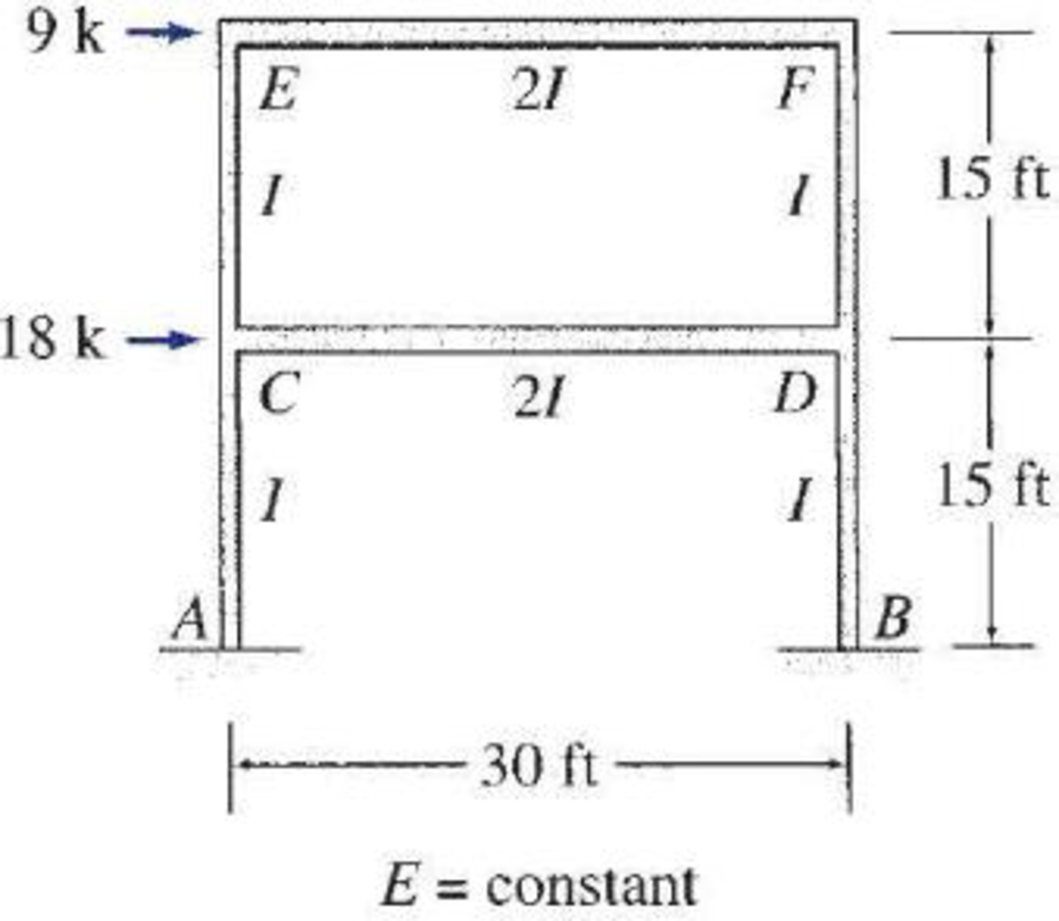# 16.24 through 16.31 Determine the member end moments and reactions for the frames shown in Figs. P16.24–P16.31 by using the moment-distribution method. FIG. P16.31

#### Solutions

Chapter
Section
Chapter 16, Problem 31P
Textbook Problem
1 views

## 16.24 through 16.31 Determine the member end moments and reactions for the frames shown in Figs. P16.24–P16.31 by using the moment-distribution method.FIG. P16.31To determine

Find the member end moments and reactions for the frames.

### Explanation of Solution

Fixed end moment:

Formula to calculate the relative stiffness for fixed support IL and for roller support (34)(IL).

Formula to calculate the fixed moment for point load with equal length are PL8.

Formula to calculate the fixed moment for point load with unequal length are Pab2L2 and Pa2bL2.

Formula to calculate the fixed moment for UDL is WL212.

Formula to calculate the fixed moment for UVL are WL230 and WL220.

Formula to calculate the fixed moment for deflection is 6EIΔL2

Calculation:

Consider the elastic modulus E of the frame is constant.

Show the free body diagram of the entire frame as in Figure 1.

Refer Figure 1,

Calculate the relative stiffness KCA, KDB, KCE, and KDF for member CA, DB, CE and DF of the frame as below:

KCA=KDB=KCE=KDF=I15

Calculate the relative stiffness KCD and KEF for member CD and EF of the frame as below:

KCD=KEF=2I30=I15

Calculate the distribution factor DFCA for member CA of the frame.

DFCA=KCAKCA+KCD+KCE

Substitute I15 for KCA, I15 for KCD, and I15 for KCE.

DFCA=I15I15+I15+I15=13

Calculate the distribution factor DFCD for member CD of the frame.

DFCD=KCDKCA+KCD+KCE

Substitute I15 for KCA, I15 for KCD, and I15 for KCE.

DFCD=I15I15+I15+I15=13

Calculate the distribution factor DFCE for member CE of the frame.

DFCE=KCEKCA+KCD+KCE

Substitute I15 for KCA, I15 for KCD, and I15 for KCE.

DFCE=I15I15+I15+I15=13

Check for sum of distribution factor as below:

DFCA+DFCD+DFCE=1

Substitute 13 for DFCA, DFCD , and DFCE.

13+13+13=1

Hence, OK.

Calculate the distribution factor DFEC for member EC of the frame.

DFEC=KECKEC+KEF

Substitute I15 for KEC and I15 for KEF.

DFEC=I15I15+I15=12

Calculate the distribution factor DFEF for member EF of the frame.

DFEF=KEFKEC+KEF

Substitute I15 for KEC and I15 for KEF.

DFEF=I15I15+I15=12

Check for sum of distribution factor as below:

DFEC+DFEF=1

Substitute 12 for DFEC and 12 for DFEF.

12+12=1

Hence, OK.

Calculate the distribution factor DFFE for member EC of the frame.

DFFE=KFEKFD+KFE

Substitute I15 for KFD and I15 for KFE.

DFFE=I15I15+I15=12

Calculate the distribution factor DFFD for member FD of the frame.

DFFD=KFDKFD+KFE

Substitute I15 for KFD and I15 for KFE.

DFFD=I15I15+I15=12

Check for sum of distribution factor as below:

DFFE+DFFD=1

Substitute 12 for DFFE and 12 for DFFD.

12+12=1

Hence, OK.

Calculate the distribution factor DFDF for member of the frame.

DFDF=KDFKDF+KDC+KDB

Substitute I15 for KDF, I15 for KDC, and I15 for KDB.

DFDF=I15I15+I15+I15=13

Calculate the distribution factor DFDC for member DC of the frame.

DFDC=KDCKDF+KDC+KDB

Substitute I15 for KDF, I15 for KDC, and I15 for KDB.

DFDC=I15I15+I15+I15=13

Calculate the distribution factor DFDB for member DB of the frame.

DFDB=KDBKDF+KDC+KDB

Substitute I15 for KDF, I15 for KDC, and I15 for KDB.

DFDB=I15I15+I15+I15=13

Check for sum of distribution factor as below:

DFDF+DFDC+DFDB=1

Substitute 13 for DFDF, 13 for DFDC, and 13 for DFDB.

13+13+13=1

Hence, OK.

Show the translation Δ1 of the frame as in figure 2.

Write the expression to calculate the Fixed-end moment of the member.

FEMAC=FEMCA=FEMBD=FEMDB=6EIΔ1(15)2

Assume the Fixed-end moment of the members AC, CA, BD and DB as 100kft.

FEMAC=FEMCA=FEMBD=FEMDB=100kft

Write the expression to calculate the Fixed-end moment of the member.

FEMCE=FEMEC=FEMDF=FEMFD=6EIΔ1(15)2

Assume the Fixed-end moment of the members CE, EC, DF and FD as 100kft.

FEMCE=FEMEC=FEMDF=FEMFD=100kft

Show the calculation of MQ1 moments using moment distribution method as in Table 1.

Show the free body diagram of the frame with unknown reaction Q21 as in Figure 3.

Consider member EC:

Calculate the horizontal reaction at the joint C by taking moment about point E.

+ME=0Cx1(15)85.261.8=0Cx1(15)=147Cx1=14715Cx1=9.8k

Calculate the horizontal reaction at joint E by resolving the horizontal equilibrium.

+Fx=0Ex1+Cx1=0Ex1+9.8=0Ex1=9.8k

Consider member FD:

Calculate the horizontal reaction at the joint D by taking moment about point F.

+MF=0Dx1(15)85.261.8=0Dx1(15)=147Dx1=14715Dx1=9.8k

Calculate the horizontal reaction at joint F by resolving the horizontal equilibrium.

+Fx=0Fx1+Dx1=0Fx1+9.8=0Fx1=9.8k

Calculate the reaction Q21 using the relation:

Q21=Ex1+Fx1=9.8+9.8=19.6k

Show the free body diagram of the frame with unknown reaction Q11 as in Figure 4.

Consider member AC:

Calculate the horizontal reaction at the joint A by taking moment about point C.

+MC=0Ax1(15)+97.1+94.2=0Ax1(15)=191.3Ax1=191.315Ax1=12.75k

Calculate the horizontal reaction at joint C by resolving the horizontal equilibrium.

+Fx=0Ax1+Cx11=0Cx1112.75=0Cx11=12.75k

Consider member BD:

Calculate the horizontal reaction at the joint B by taking moment about point D.

+MD=0Bx1(15)+94.2+97.1=0Bx1(15)=191.3Bx1=191.315Bx1=12.75k

Calculate the horizontal reaction at joint D by resolving the horizontal equilibrium.

+Fx=0Bx1+Dx11=012

### Still sussing out bartleby?

Check out a sample textbook solution.

See a sample solution

#### The Solution to Your Study Problems

Bartleby provides explanations to thousands of textbook problems written by our experts, many with advanced degrees!

Get Started

Find more solutions based on key concepts
Present Example 12.4 in Chapter 12 using the format discussed in Section 4.2. Divide the example problem into G...

Engineering Fundamentals: An Introduction to Engineering (MindTap Course List)

What is the purpose of layout fluid (dye)?

Precision Machining Technology (MindTap Course List)

Describe security risks associated with wireless access. Identify ways to secure your wireless network.

Enhanced Discovering Computers 2017 (Shelly Cashman Series) (MindTap Course List)

Describe how a Wi-Fi network works.

Principles of Information Systems (MindTap Course List)

What is XML, and how is it used?

Fundamentals of Information Systems

Name two advantages of recycling scrap metal.

Welding: Principles and Applications (MindTap Course List)

If your motherboard supports ECC DDR3 memory, can you substitute non-ECC DDR3 memory?

A+ Guide to Hardware (Standalone Book) (MindTap Course List)